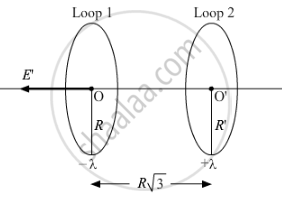# Find the Magnitude and Direction of the Net Electric Field at the Centre of Loop '1'. - Physics

Numerical
Short Note

Two identical circular loops 1 and 2 of radius R each have linear charge densities −λ and +λ C/m respectively. The loops are placed coaxially with their centres Rsqrt3 distance apart. Find the magnitude and direction of the net electric field at the centre of loop 1.

#### Solution

Magnitude of electric field at any point on the axis of a uniformly charged loop is given by,

E=λ/(2∈_0) (rR)/(r^2+R^2)^(3/2)  .....(i)

where
R = Radius of the loop
r = Position of the point from the centre of the loop
λ = Linear charge density of the loopElectric field at the centre of loop 1 due to charge present on it is zero. [ From (i), when r = 0 ]
| vecE_1 | = 0 (As Z = 0)

Electric field at a point outside the loop 2 on the axis passing normally is
|vecE_2| = [lambdaR]/(2ε_0) . [Z]/[R^2 + Z^2]^(3/2)

Since,                Z = Rsqrt3

= [lambdaR]/(2ε_0) . [Rsqrt3]/[(R^2 + 3R^2)^(3/2)]

= [lambdasqrt3]/[16ε_0R]     twowards right (As λ is positive)

So, net electric field at the centre of loop 1

vecE = vecE_1 + vecE_2

= 0 + [lambdasqrt3]/[16ε_0R] = [lambdasqrt3]/[16ε_0R]

This is the net electric field at the centre of loop 1 due to the charge on both the loops. The direction of this net field is from loop 2 to loop 1 as shown in the above figure.

Concept: Electric Field - Introduction of Electric Field
Is there an error in this question or solution?
2014-2015 (March) Patna Set 2
Share